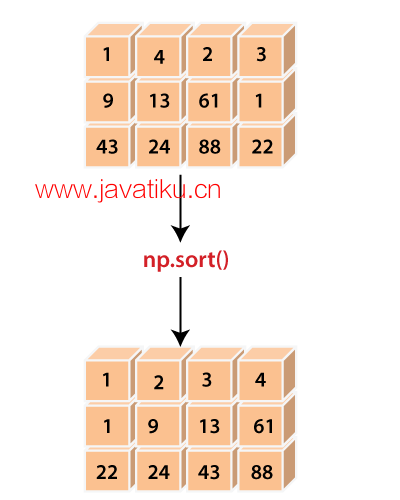# NumPy教程-numpy.sort()在Python中的使用### 语法：

``numpy.sort(a, axis=-1, kind='quicksort', order=None)``

### 参数：

x: array_like

axis: int或None（可选）

kind: {quicksort, heapsort, mergesort}（可选）

order: str或str列表（可选）

### 示例1：

``````import numpy as np
x=np.array([[1,4,2,3],[9,13,61,1],[43,24,88,22]])
x
y=np.sort(x)
y  ``````

``````array([[ 1,  4,  2,  3],
[ 9, 13, 61,  1],
[43, 24, 88, 22]])
array([[ 1,  2,  3,  4],
[ 1,  9, 13, 61],
[22, 24, 43, 88]])``````

• 我们导入了别名为np的numpy。
• 我们使用np.array()函数创建了一个多维数组'x'
• 我们声明了变量'y'，并将np.sort()函数的返回值赋值给它。
• 我们在函数中传递了输入数组'x'
• 最后，我们尝试打印'y'的值。

### 示例2：

``````import numpy as np
x=np.array([[1,4,2,3],[9,13,61,1],[43,24,88,22]])
x
y=np.sort(x, axis=None)
y  ``````

``````array([[ 1,  4,  2,  3],
[ 9, 13, 61,  1],
[43, 24, 88, 22]])
array([ 1,  1,  2,  3,  4,  9, 13, 22, 24, 43, 61, 88])``````

### 示例3：

``````import numpy as np
x=np.array([[1,4,2,3],[9,13,61,1],[43,24,88,22]])
x
y=np.sort(x,axis=0)
y
z=np.sort(x,axis=1)
z  ``````

``````array([[ 1,  4,  2,  1],
[ 9, 13, 61,  3],
[43, 24, 88, 22]])
array([[ 1,  2,  3,  4],
[ 1,  9, 13, 61],
[22, 24, 43, 88]])``````

### 示例4：

``````import numpy as np
dtype = [('name', 'S10'), ('height', float), ('age', int),('gender','S10')]
values = [('Shubham', 5.9, 23, 'M'), ('Arpita', 5.6, 23, 'F'),('Vaishali', 5.2, 30, 'F')]
x=np.array(values, dtype=dtype)
x
y=np.sort(x, order='age')
y
z=np.sort(x, order=['age','height'])
z  ``````

``````array([('Shubham', 5.9, 23, 'M'), ('Arpita', 5.6, 23, 'F'), ('Vaishali', 5.2, 30, 'F')],dtype=[('name', 'S10'), ('height', '<f8'), ('age', '<i4'), ('gender', 'S10')])
array([('Arpita', 5.6, 23, 'F'), ('Shubham', 5.9, 23, 'M'), ('Vaishali', 5.2, 30, 'F')], dtype=[('name', 'S10'), ('height', '<f8'), ('age', '<i4'), ('gender', 'S10')])
array([('Arpita', 5.6, 23, 'F'), ('Shubham', 5.9, 23, 'M'), ('Vaishali', 5.2, 30, 'F')], dtype=[('name', 'S10'), ('height', '<f8'), ('age', '<i4'), ('gender', 'S10')])``````

• 我们导入了别名为np的numpy。
• 我们定义了结构化数组的字段和值。
• 我们通过在np.array()函数中传递dtype和values来创建了一个结构化数组'x'
• 我们声明了变量'y''z'，并将np.sort()函数的返回值赋值给它们。
• 我们在函数中传递了输入数组'x'和order。
• 最后，我们尝试打印'y''z'的值。